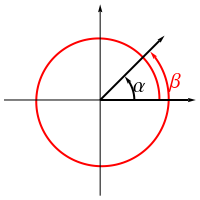# Get Tutoring Info Now!

(800) 434-2582

/ Get Math Help

## Coterminal Angles

IllustrationDefinition

Two non-coincident plane angles α and β in angle standard position are said to be coterminal if the terminal side of α is identically the same as the terminal side of β. In general, given a plane angle α measured in radians, β is coterminal to α if and only if β = α ± 2n π for some positive integer n element Z^+. Similarly, if β is a plane angle coterminal to a plane angle α measured in degrees, then β = α ± 360n for some positive integer n element Z^+. In the event that n = 0, then α and β are coincident.

Related terms